# Difference between revisions of "SOCR EduMaterials Activities PowerTransformFamily Graphs"

## Summary

This is activity demonstrates the usage, effects and properties of the modified power transformation family applied to real or simulated data to reduce variation and enhance Normality. There are 4 exercises each demonstrating the properties of the power transform in different settings for observed or simulated data: X-Y scatter plot, QQ-Normal plot, Histogram plot and Time/Index plot.

## Background

The power transformation family is often used for transforming data for the purpose of making it more Normal-like. The power transformation is continuously varying with respect to the power parameter $$\lambda$$ and defined, as continuous piece-wise function, for all $$y>0$$ by

$$y^{(\lambda)} = \left\{ \begin{matrix} {(y^{\lambda}-1) \over {\lambda} } & \mathrm{for\ } \lambda \neq 0 \\ & \\ \log{y} & \mathrm{for\ } \lambda = 0 \end{matrix} \right.$$

## Exercises

### Exercise 1: Power Transformation Family in a X-Y Scatter Plot Setting

• This exercise demonstrates the characteristics of the power-transform when applied independently to the two processes in an X-Y scatter plot setting. In this situation, one observes paired (X,Y) values which are typically plotted X vs. Y in the 2D plane. We are interested in studying the effects of independently applying the power transforms to the X and Y processes. How and why would the corresponding scatter plot change as we vary the power parameters for X and Y?
• First, point your browser to SOCR Charts and select the PowerTransformXYStatterChart (Line-Charts -> PowerTransformXYStatterChart). You may use the default data provided for this chart, enter your own data (remember to MAP the data before your UPDATE the chart), or obtain SOCR simulated data from the Data-Generation tab of the SOCR Modeler (an example is shown later in Exercise 4). As shown on the image below, try changing the power parameters for the X and Y power-transforms and observe the graphical behavior of the transformed scatter-plot (blue points connected by a thin line) versus the native (original) data (red color points). We have applied a linear rescaling to the power-transform data to map it in the same space as the original data. This is done purely for visualization purposes, as without this rescaling it will be difficult to see the correspondence of the transformed and original data. Also note the changes of the numerical summaries for the transformed data (bottom text area) as you update the power parameters. What power parameters would you suggest that make the X-Y relation most linear?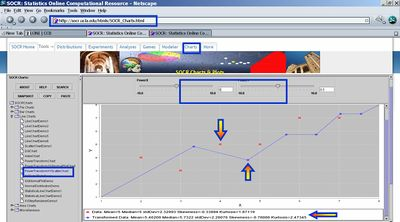### Exercise 2: Power Transformation Family in a QQ-Normal Plot Setting

• The second exercise demonstrates the effects of the power-transform applied to data in a QQ-Normal plot setting. We are interested in studying the effects of power transforming the native (original) data on the quantiles, relative the Normal quantiles (i.e., QQ-Normal plot effects). How and why do you expect the QQ-Normal plot to change as we vary the power parameter?
• Again go to SOCR Charts and select the PowerTransformQQNormalPlotChart (Line-Charts -> PowerTransformQQNormalPlotChart). You can use different data for this experiment - either use the default data provided with the QQ-Normal chart, enter your own data (remember to MAP the data before your UPDATE the chart) or obtain SOCR simulated data from the Data-Generation tab of the SOCR Modeler (an example is shown later in Exercise 4). Change the power-transform parameter (using the slider or the by typing in the text area) and observe the graphical behavior of the transformed data in the QQ-Normal plot (green points connected by a thin line) versus the plot of the native data (red color points). What power parameter would you suggest that make the (transformed) data quantiles similar to those of the Normal distribution? Why?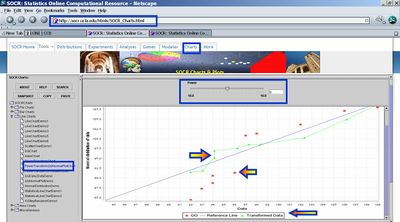### Exercise 3: Power Transformation Family in a Histogram Plot Setting

• This exercise demonstrates the effects on the histogram distribution after applying the power-transform to the (observed or simulated) data. In this experiment, we want to see whether we can reduce the variance of a dataset and make its histogram more symmetric, unimodal and bell-shaped.
• Again go to SOCR Charts and select the PowerTransformHistogramChart (Bar-Charts -> XYPlot -> PowerTransformHistogramChart). We will use SOCR simulated data from the Data-Generation tab of the SOCR Modeler, however you may choose to use the default data for this chart or enter your own data. The image below shows you the Generalized Beta Distribution using SOCR Distributions.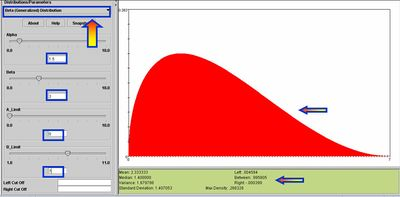• Go to the SOCR Modeler and select 200 observations from the Generalized Beta Distribution ($$\alpha = 1.5; \beta = 3; A=0; B=7$$), as shown on the image below. Copy these 200 values in your mouse buffer (CNT-C) and paste them in the Data tab of the PowerTransformHistogramChart. Then map this column to XYValue (under the MAP tab) and click Update_Chart. This will generate the histogram of the 200 observations. Indeed, this graph should look like a discrete analog of the Generalized Beta density curve above.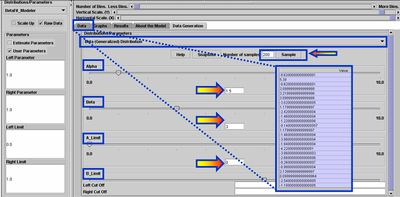• In the Graph tab of the PowerTransformHistogramChart, change the power-transform parameter (using the slider on the top). All SOCR Histogram charts allow you to choose the width of the histogram bins, using the second slider on the top. Observe the graphical behavior of the histogram of the transformed data (blue bins) and compare it to the histogram of the native data (red bins). What power parameter would you suggest that make the histogram of the power-transformed data better? Why?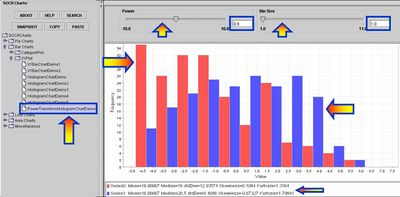### Exercise 4: Power Transformation Family in a Time/Index Plot Setting

• Let’s first get some data: Go to SOCR Modeler and generate 100 Cauchy Distributed variables. Copy these data in your mouse buffer (CNT-C). Of course, you may use your own data throughout. We choose Cauchy data to demonstrate how the Power Transform Family allows us to normalize data that is far from being Normal-like.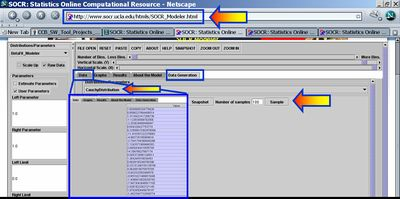• Next, paste (CNT-V) these 100 observations in SOCR Charts (Line-Charts -> Power Transform Chart). Click Update Chart to see the index plot of this data in RED!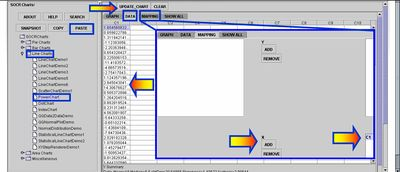• Now go to the Graph Tab-Pane and choose $$\lambda = 0$$ (the power parameter). Why is $$\lambda = 0$$ the best choice for this data? Try experimenting with different values of $$\lambda$$. Observe the variability in the Graph of the transformed data in Blue (relative to the variability of the native data in Red).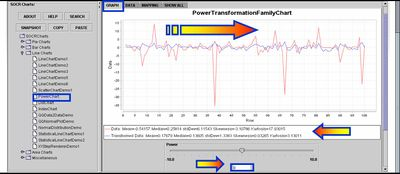• Then go back to the Data Tab-Pane and copy in your mouse buffer the transformed data. We will compare how well does Normal distribution fit the histograms of the raw data ( Cauchy distribution) and the transformed data. One can experiment with other powers of $$\lambda$$, as well! In the case of $$\lambda =0$$, the power transform reduces to a log transform, which is generally a good way to make the histogram of a data set well approximated by a Normal Distribution. In our case, the histogram of the original data is close to Cauchy distribution, which is heavy tailed and far from Normal (Recall that the T(df) distribution provides a 1-parameter homotopy between Cauchy and Normal).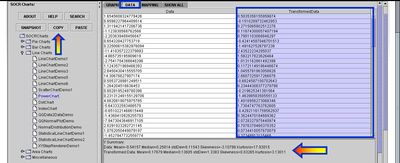• Now copy in your mouse buffer the transformed data and paste it in the SOCR Modeler. Check the Estimate Parameters check-box on the top-left. This will allow you to fit a Normal curve to the histogram of the (log) Power Family Transformed Data. You see that Normal Distribution is a great fit to the histogram of the transformed Data. Be sure to check the parameters of the Normal Distribution (these are estimated using least squares and reported in the Results Tab-Pane). In this case, these parameters are: Mean = 0.177, Variance = 1.77, however, these will vary, in general.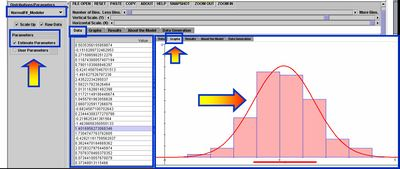• Let’s try to fit a Normal model to the histogram of the native data (recall that this histogram should be shaped as Cauchy, as we sampled from Cauchy distribution – therefore, we would not expect a Normal Distribution to be a good fit for these data. This fact, by itself, demonstrates the importance of the Power Transformation Family. Basically we were able to Normalize a significantly Non-Normal data set. Go back to the original SOCR Modeler, where you sampled the 100 Cauchy observations. Select NormalFit_Modeler from the drop-down list of models in the top-left and click on the Graphs and Results Tab-Panes to see the graphical results of the histogram of the native (heavy-tailed) data and the parameters of its best Normal Fit. Clearly, as expected, we do not have a got match.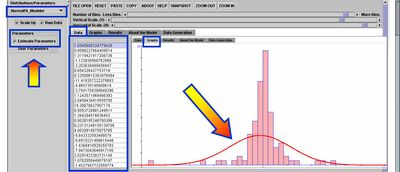• Questions
• Try experimenting with other (real or simulated) data sets and different Power parameters (λ). What are the general effects of increasing/decreasing λ in any of these domains [-10;0], [0;1] and [1;10]?
• For each of the exercises (X-Y scatter-plot, QQ-Normal plot, Histogram plot and Time/Index plot) empirically study the effects of the power transform as a tool for normalizing the data. You can take samples of size 100 from Student’s T-distribution (low df) and determine appropriate levels of λ for which the transformed data is (visually) well approximated by a Normal Distribution.